# Tejero and Cuesta hard disk equation of state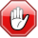This page contains numerical values and/or equations. If you intend to use ANY of the numbers or equations found in SklogWiki in any way, you MUST take them from the original published article or book, and cite the relevant source accordingly.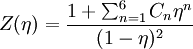$Z (\eta)= \frac{1+\sum_{n=1}^6 C_n \eta^n}{(1-\eta)^2}$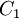$C_1$ 0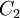$C_2$ 0.128018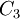$C_3$ 0.0018188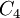$C_4$ -0.72553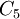$C_5$ 2.52783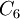$C_6$ -2.4945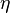$\eta$ is the packing fraction and Z is the compressibility factor.